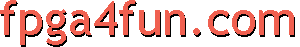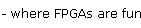## Music box siren ramp

 Let me make an analogy. Let's say I have a 6 digits decimal counter that goes from 000000 to 199999. The counter is free counting, increments every 1 millisecond (i.e. 1000 times a second) and after it reaches 199999, it returns to 0 and keeps going up again. At that speed, this counter rolls over every 200 seconds. Obviously, the first digit (I call it digit #0, or digit) will increment the fastest. Too fast for your eyes, so unusable. But the second digit (digit #1) will increment 10 times slower. And so on... Now let's say you look only at digits #4 and #3 (=digit[4:3]). The other digits are still going on at their respective speeds; you are just not looking at them. You'll see digit[4:3] forming a number between 0 and 99, and incrementing every second. Furthermore, I make the following rule: if digit is '0', the I look at digit[4:3], while if digit #5 is '1', I look at the opposite, i.e. (99-digit[4:3]). In other words, if(digit=='0') then my rampvalue=digit[4:3] else if(digit=='1') then my rampvalue=(99-digit[4:3]); That gives me a ramp going up from 0 to 99 and then down from 99 to 0, and then again from 0 to 99... ``` digits[5:3] rampvalue 000 00 001 01 ... .. ... .. 098 98 099 99 100 99 101 98 102 97 103 96 ... .. ... .. 197 02 198 01 199 00 000 00 001 01 002 02 ... .. ```